# Solving Trigonometric Equations Part 1 Equations that need

• Slides: 17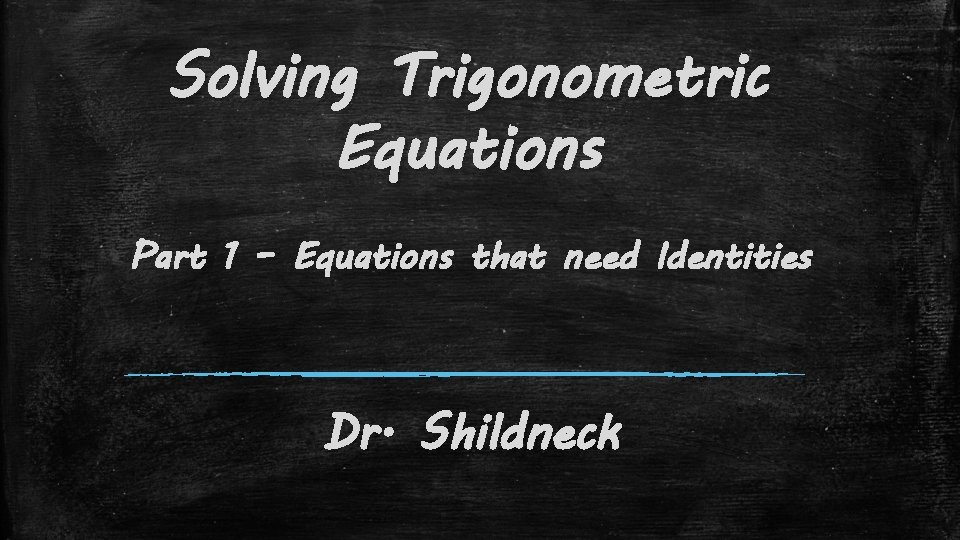Solving Trigonometric Equations Part 1 – Equations that need Identities Dr. ShildneckDealing with “tricky” equations ▪ Use a trig identity to alter the equation; simplify ▪ Solve the equation to isolate the trig function ▪ Solve the trig function for the variable ▪ Verify answers in original equationExample 1 ▪ Solve the equation on the interval [0, 2π).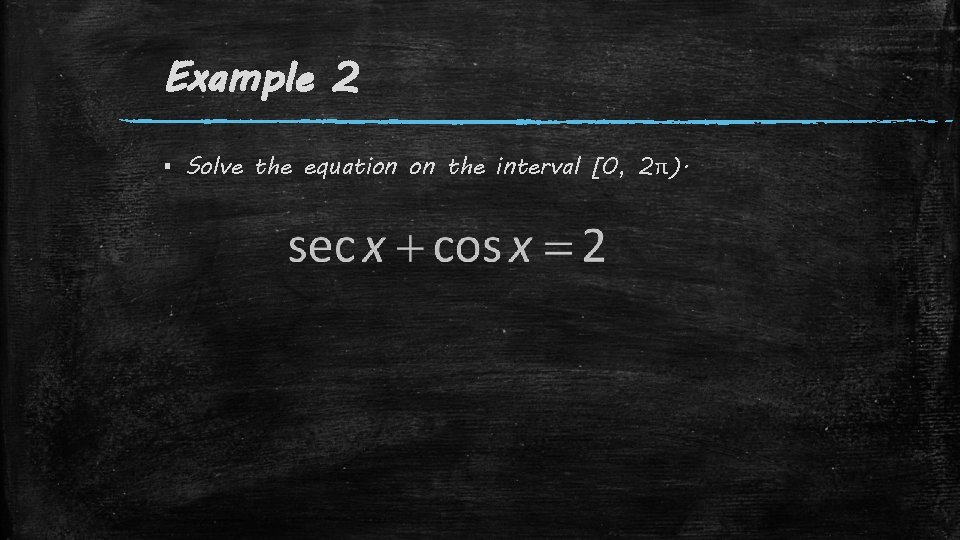Example 2 ▪ Solve the equation on the interval [0, 2π).Solving Trigonometric Equations Part 2 - Equations with “Multiple Angles” Dr. Shildneck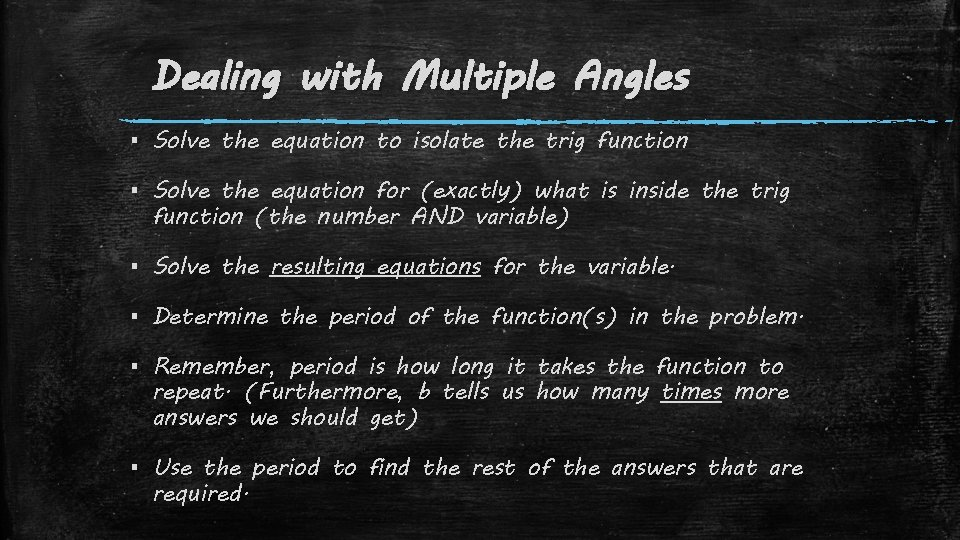Dealing with Multiple Angles ▪ Solve the equation to isolate the trig function ▪ Solve the equation for (exactly) what is inside the trig function (the number AND variable) ▪ Solve the resulting equations for the variable. ▪ Determine the period of the function(s) in the problem. ▪ Remember, period is how long it takes the function to repeat. (Furthermore, b tells us how many times more answers we should get) ▪ Use the period to find the rest of the answers that are required.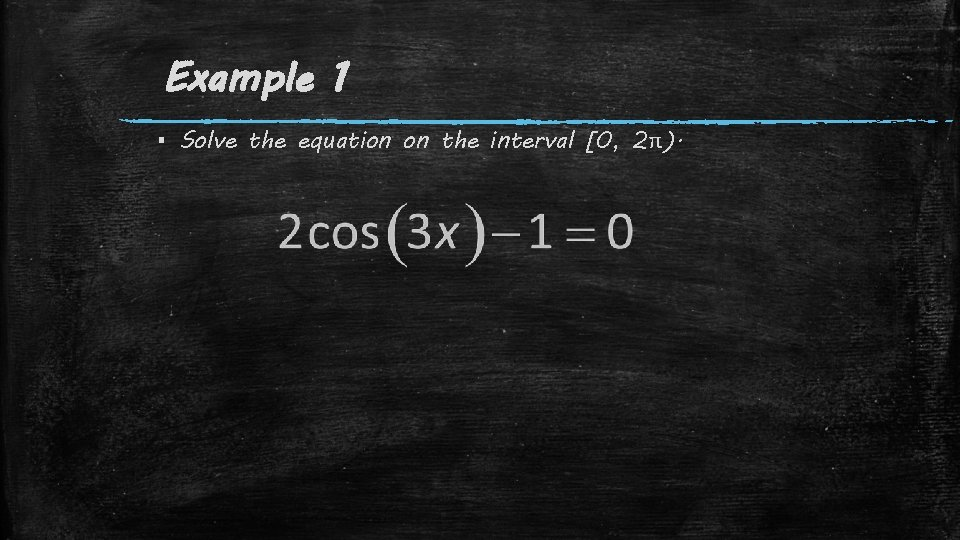Example 1 ▪ Solve the equation on the interval [0, 2π).Example 2 ▪ Solve the equation on the interval [0, 2π).Example 3 ▪ Solve the equation on [0, 2π).Example 4 ▪ Solve the equation on [0, 2π).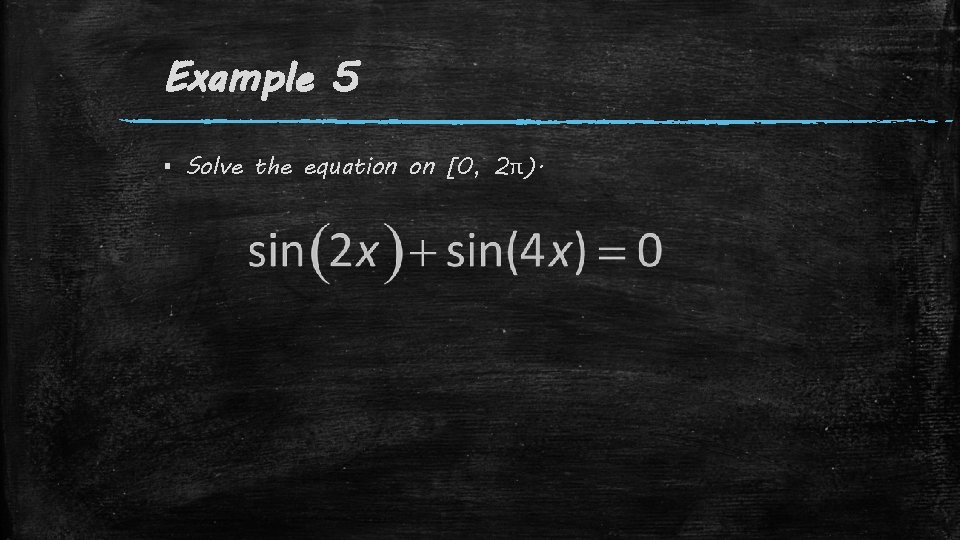Example 5 ▪ Solve the equation on [0, 2π).Solving Trigonometric Equations Part 3 - Non-Standard Values Dr. Shildneck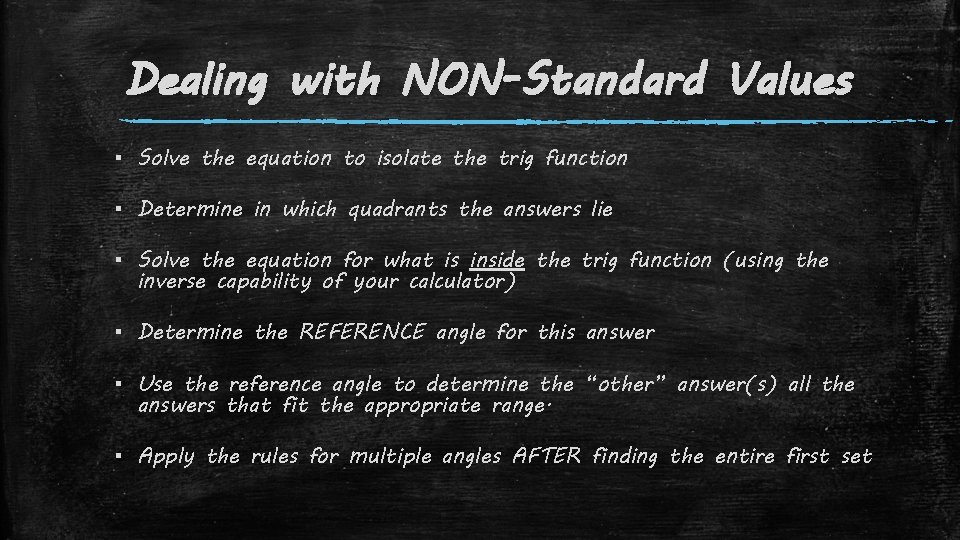Dealing with NON-Standard Values ▪ Solve the equation to isolate the trig function ▪ Determine in which quadrants the answers lie ▪ Solve the equation for what is inside the trig function (using the inverse capability of your calculator) ▪ Determine the REFERENCE angle for this answer ▪ Use the reference angle to determine the “other” answer(s) all the answers that fit the appropriate range. ▪ Apply the rules for multiple angles AFTER finding the entire first set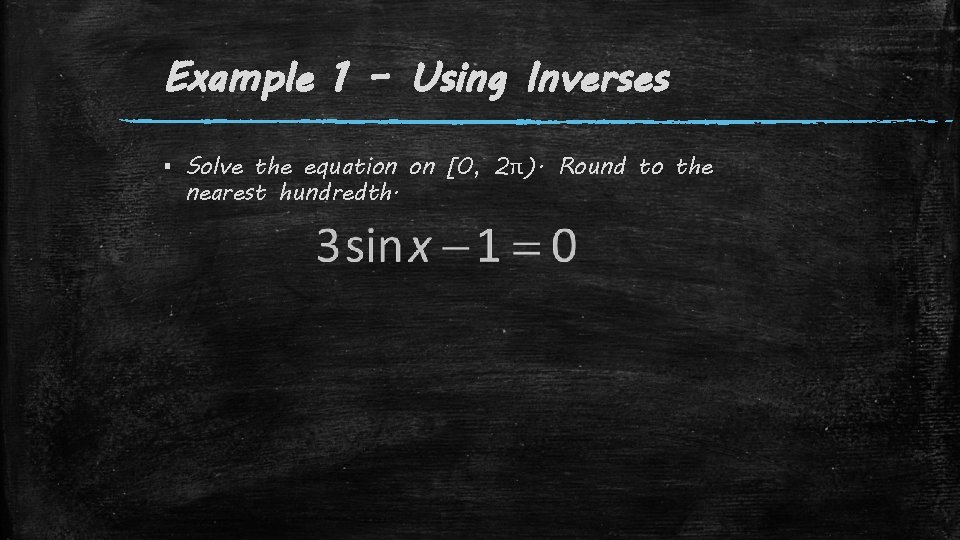Example 1 – Using Inverses ▪ Solve the equation on [0, 2π). Round to the nearest hundredth.Example 2 – Using Inverses ▪ Solve the equation on [0, 2π). Round to the nearest hundredth.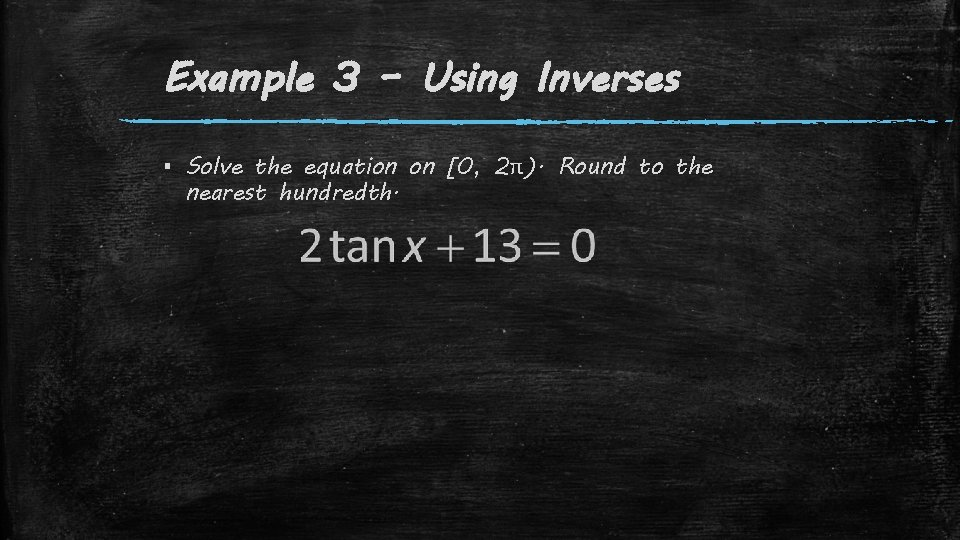Example 3 – Using Inverses ▪ Solve the equation on [0, 2π). Round to the nearest hundredth.Example 4 – Using Inverses ▪ Solve the equation on [0, 2π). Round to the nearest hundredth.